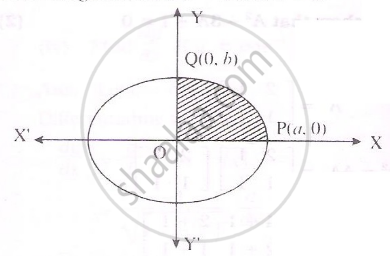HSC Commerce 12th Board ExamMaharashtra State Board
Share

Books Shortlist
Your shortlist is empty

# Solution for Find the area of elipse x^2/a^2+y^2/b^2=1 - HSC Commerce 12th Board Exam - Mathematics and Statistics

ConceptApplications of Definite Integrals

#### Question

Find the area of elipse x^2/a^2+y^2/b^2=1

#### Solution

equation of elipse x^2/a^2+y^2/b^2=1

Clearly the area of elipse is 4 times the area of region OPQD as show in the figure.For the region.Limits of integration are x=0 and x=aFrom the elipse

y^2/b^2=1-x^2/a^2=(a^2-x^2)/a^2

therefore y^2=b^2/a^2(a^2-x^2)

y=+-b/a(a^2-x^2)^(1/2)

y=b/a(a^2-x^2)^(1/2)

We know

A=4int_0^1ydx

=int_0^ab/a(a^2-x^2)^(1/2)dx

=(4b)/a[x/2(a^2-x^2)^(1/2)+a^2/2sin^(-1)(x/a)]_0^a

=(4b)/a[a^2/2 pi/2-0]

=ab pi

Is there an error in this question or solution?

#### APPEARS IN

2014-2015 (March) (with solutions)
Question 2.2.2 | 4.00 marks

#### Video TutorialsVIEW ALL 

Solution for question: Find the area of elipse x^2/a^2+y^2/b^2=1 concept: Applications of Definite Integrals. For the courses HSC Commerce, HSC Commerce (Marketing and Salesmanship)
S